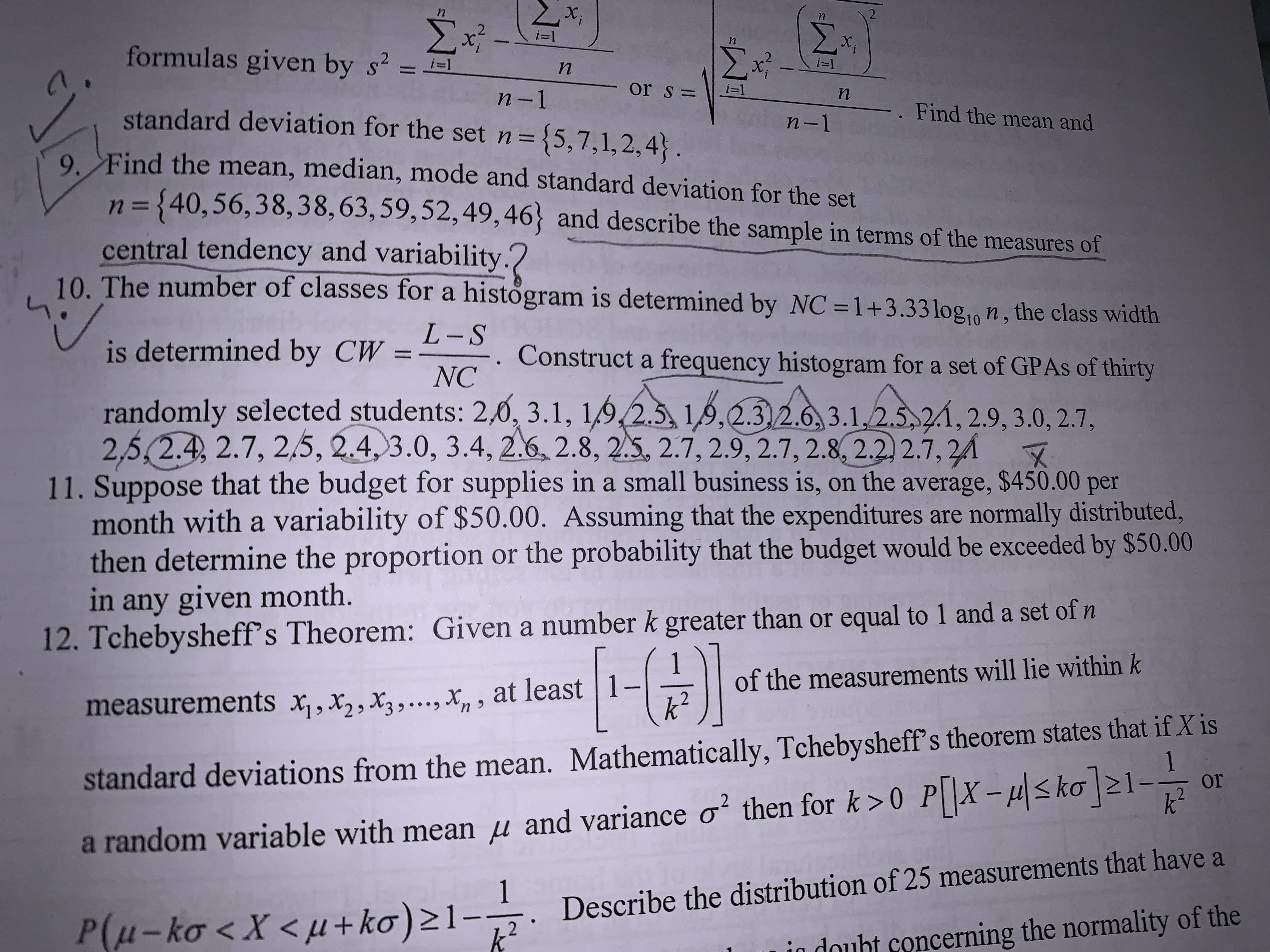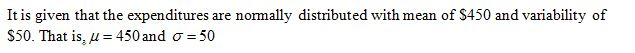# M-Σ.formulas given by s² =ΣΣi=1х,i=1i=1n-1or sDi=1standard deviation for the set n={5,7,1,2,4}.-. Find the mean andn-19. Find the mean, median, mode and standard deviation for the setn 3=n={40,56,38,38,63,59,52,49,46} and describe the sample in terms of the measures ofcentral tendency and variability.?10. The number of classes for a histögram is determined by NC =1+3.33 log,, n, the class widthL-Sis determined by CW =NCConstruct a frequency histogram for a set of GPAS of thirtyrandomly selected students: 2,0, 3.1, 1,9,2.5, 1,9,2.3,2.6, 3.1,2.5,2.1, 2.9, 3.0, 2.7,2,5,2.4, 2.7, 2,5, 2.4, 3.0, 3.4, 2.6, 2.8, 2.5, 2.7, 2.9, 2.7, 2.8, 2.2) 2.7, 2411. Suppose that the budget for supplies in a small business is, on the average, \$450.00 permonth with a variability of \$50.00. Assuming that the expenditures are normally distributed,then determine the proportion or the probability that the budget would be exceeded by \$50.00in any given month.12. Tchebysheff's Theorem: Given a number k greater than or equal to 1 and a set of n| 1-k2of the measurements will lie within kmeasurements x,, x,,X3,.….., X, , at leaststandard deviations from the mean. Mathematically, Tchebysheff's theorem states that if X isork²a random variable with mean µ and variance o² then for k >0 P X-µ

Question
3 views

Question 11help_outlineImage TranscriptioncloseM- Σ. formulas given by s² = Σ Σ i=1 х, i=1 i=1 n-1 or sD i=1 standard deviation for the set n={5,7,1,2,4}. -. Find the mean and n-1 9. Find the mean, median, mode and standard deviation for the set n 3= n={40,56,38,38,63,59,52,49,46} and describe the sample in terms of the measures of central tendency and variability.? 10. The number of classes for a histögram is determined by NC =1+3.33 log,, n, the class width L-S is determined by CW = NC Construct a frequency histogram for a set of GPAS of thirty randomly selected students: 2,0, 3.1, 1,9,2.5, 1,9,2.3,2.6, 3.1,2.5,2.1, 2.9, 3.0, 2.7, 2,5,2.4, 2.7, 2,5, 2.4, 3.0, 3.4, 2.6, 2.8, 2.5, 2.7, 2.9, 2.7, 2.8, 2.2) 2.7, 24 11. Suppose that the budget for supplies in a small business is, on the average, \$450.00 per month with a variability of \$50.00. Assuming that the expenditures are normally distributed, then determine the proportion or the probability that the budget would be exceeded by \$50.00 in any given month. 12. Tchebysheff's Theorem: Given a number k greater than or equal to 1 and a set of n | 1- k2 of the measurements will lie within k measurements x,, x,,X3,.….., X, , at least standard deviations from the mean. Mathematically, Tchebysheff's theorem states that if X is or k² a random variable with mean µ and variance o² then for k >0 P X-µ
check_circle

Step 1

Note: The given variability is considered as standard deviation.

Step 2...

### Want to see the full answer?

See Solution

#### Want to see this answer and more?

Solutions are written by subject experts who are available 24/7. Questions are typically answered within 1 hour.*

See Solution
*Response times may vary by subject and question.
Tagged in